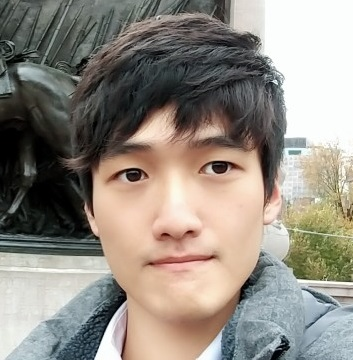Jaewoo Song

### Categories

• Tech

Sequence-to-Sequence(seq2seq) model is a Deep Learning model usually used for machine translation, text summarization etc.

As we can see from its name, a seq2seq model takes a sequence as an input and gives us a different sequence as an output.

With the attention mechanism, this model became the basis of the famous Transformer model, so let me post about this before moving on to the Attention mechanism.

First, this is the basic architecture of a seq2seq model.

Basically, it consists of two main parts, the Encoder and the Decoder.

Let us assume that we conduct a machine translation task.Above picture shows the training mechanism with a seq2seq model for machine translation.

Both the original sentence and the translated one become inputs.

After tokenized, each token is converted into an embedded vector through the embedding layer.

As we can see, the translated input sentence is not just put into the model.

Each token which constitutes that sentence is pushed back one spot and additional “sos(start of sentence)” token is put in front of the translated sentence.

Now, the encoder LSTM takes the original input and makes it into a context vector, which contains overall context of the given input.

This is actually a last hidden state from the encoder LSTM so its size is same with the hidden size of the encoder LSTM.

Then the context vector is put into the decoder as the first hidden state of the decoder LSTM.

By taking the translated input, the decoder makes outputs and these are compared with the correctly translated input.

To be more specific, the decoder is trained as if it takes the context vector and additional input $(start \to tokA \to tokB \to tokC)$, then it should generate the correct output which is $(tokA \to tokB \to tokC \to end)$.

The “eos” token stands for the end of sentence.

This is called “Teaching forcing” which the real sentence is put into RNN based model to help it to predict correct answer and to be trained faster.

If the predicted output of one LSTM cell is put into the next cell as an input, then it makes much harder for the entire model to be trained correctly, which leads to the degradation of overall performances.

Of course when testing we don’t know the correct answer, so the predicted value from one decoder cell is put into the next cell as an input.

Back to the training procedure, each decoder cell gives an output hidden vector and these outputs go through a fully connected layer, which has a size of $h \times vocab\_size$. ($h$ is hidden size and $vocab\_size$ is the size of vocab.)

Then after the softmax, it is same with a token level classification task.(Calculating loss, backpropagation….etc.)

Now let’s see how this model works in the testing phase.As I mentioned above, we don’t know the correct translated sequence in testing.

Therefore, we should put the output from the previous LSTM cell into the next cell as an input.

Like the training phase, after the fully connected layer and the softmax, it chooses the most likely word in the vocab and makes the output sequence.

I have been explaining the overall process assuming a translation task, but with the same mechanism we can use it various NLG tasks, such as text summarization, question-answering system, chatbot etc.

So this is the overall understanding of the seq2seq model.

Since the advent of Transformer, the usage of this model has decreased but it is important to know it to understand the structure of Transformer.

Next time, I will post about another very important part in NLP, the attention mechanism.

1) 시퀀스-투-시퀀스(Sequence-to-Sequence, seq2seq). (2020, Mar 10). https://wikidocs.net/24996.